# Connectedness is not weakly hereditary

This article gives the statement, and possibly proof, of a topological space property (i.e., connected space) not satisfying a topological space metaproperty (i.e., weakly hereditary property of topological spaces).
View all topological space metaproperty dissatisfactions | View all topological space metaproperty satisfactions|Get help on looking up metaproperty (dis)satisfactions for topological space properties
Get more facts about connected space|Get more facts about weakly hereditary property of topological spaces|

## Statement

It is possible to have a nonempty topological space$X$ and a nonempty closed subset$A$ of$X$ such that:

•$X$ is a connected space
•$A$ is not a connected space under the subspace topology from$X$.

## Proof

### Example using finite topological spaces

For a counterexample,$A$ must have at least two points, because the unique one-point space is connected. Therefore,$X$ must have at least three points. Below is one such example: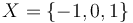$X = \{ -1, 0, 1 \}$

with the topology defined as follows: the open subsets are: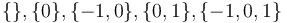$\{ \}, \{ 0 \}, \{ -1, 0 \}, \{ 0, 1 \}, \{ -1, 0, 1 \}$

Or equivalently, the closed subsets are: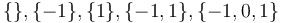$\{ \}, \{ -1 \}, \{ 1 \}, \{ -1, 1 \}, \{ -1, 0, 1 \}$

Clearly,$X$ is connected: the only nonempty closed subset containing$0$ is all of$X$, and therefore$X$ cannot be expressed as a union of two disjoint nonempty open subsets. In fact, framed more strongly,$X$ is an irreducible space.

Consider$A$ to be the subset$\{ -1, 1 \}$ of$X$ with the subspace topology.$A$ has a discrete topology, and in particular, is a union of disjoint closed subsets$\{ -1 \}$ and$\{ 1 \}$. Therefore, it is not connected.

Basically, the point$0$ serves the role of connecting the space, and removing it disconnects the space.

### Example using the real line and a finite subset

Consider the following example:

•$X = \R$ is the set of real numbers endowed with the usual Euclidean topology.
•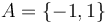$A = \{ -1, 1 \}$ is a subset of size two.$X$ is connected.$A$ is discrete in the subspace topology. Explicitly, for instance,$\{-1 \}$ is the intersection of$A$ with the open subset$(-\infty, 0)$ of$X$, hence is open in$A$, and similarly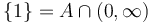$\{ 1 \} = A \cap (0, \infty)$ and hence is open in$A$.